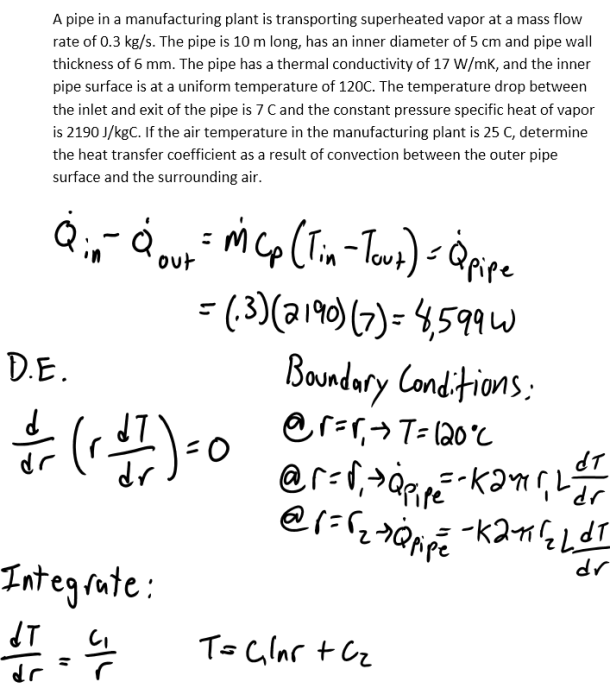heat and mass transfer problems engineering equations heat and mass transfer school homework engineering heat and mass transfer formulas heat and mass transfer solutions to heat and mass transfer problems full solution engineering problem solution heat and mass transfer math problems engineering equations heat and mass transfer school homework engineering solutions to heat and mass transfer formulas heat problems mass problem solutions to transfer problems full solution heat and mass transfer
heat and mass transfer problems engineering equations heat and mass transfer school homework engineering heat and mass transfer formulas heat and mass transfer solutions to heat and mass transfer problems full solution engineering problem solution heat and mass transfer math problems engineering equations heat and mass transfer school homework engineering solutions to heat and mass transfer formulas heat problems mass problem solutions to transfer problems full solution heat and mass transfer
Highalphabet Home Page heat and mass transfer problem solutions Heat and Mass Transfer Page
A pipe in a manufacturing plant is transporting superheated vapor at a mass flow rate of 0.3 kg/s. The pipe is 10 m long, has an inner diameter of 5 cm and pipe wall thickness of 6 mm. The pipe has a thermal conductivity of 17 W/mK, and the inner pipe surface is at a uniform temperature of 120 C. The temperature drop between the inlet and exit of the pipe is 7 C and the constant pressure specific heat of vapor is 2190 J/kgC. If the air temperature in the manufacturing plant is 25 C, determine the heat transfer coefficient as a result of convection between the outer pipe surface and the surrounding air.A pipe in a manufacturing plant is transporting superheated vapor at a mass flow rate of 0.3 kg/s. The pipe is 10 m long, has an inner diameter of 5 cm and pipe wall thickness of 6 mm. The pipe has a thermal conductivity of 17 W/mK, and the inner pipe surface is at a uniform temperature of 120 C. The temperature drop between the inlet and exit of the pipe is 7 C and the constant pressure specific heat of vapor is 2190 J/kgC. If the air temperature in the manufacturing plant is 25 C, determine the heat transfer coefficient as a result of convection between the outer pipe surface and the surrounding air.